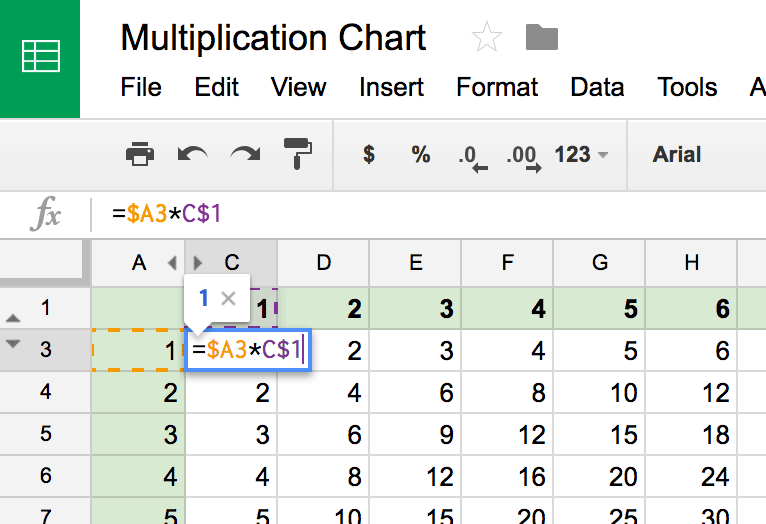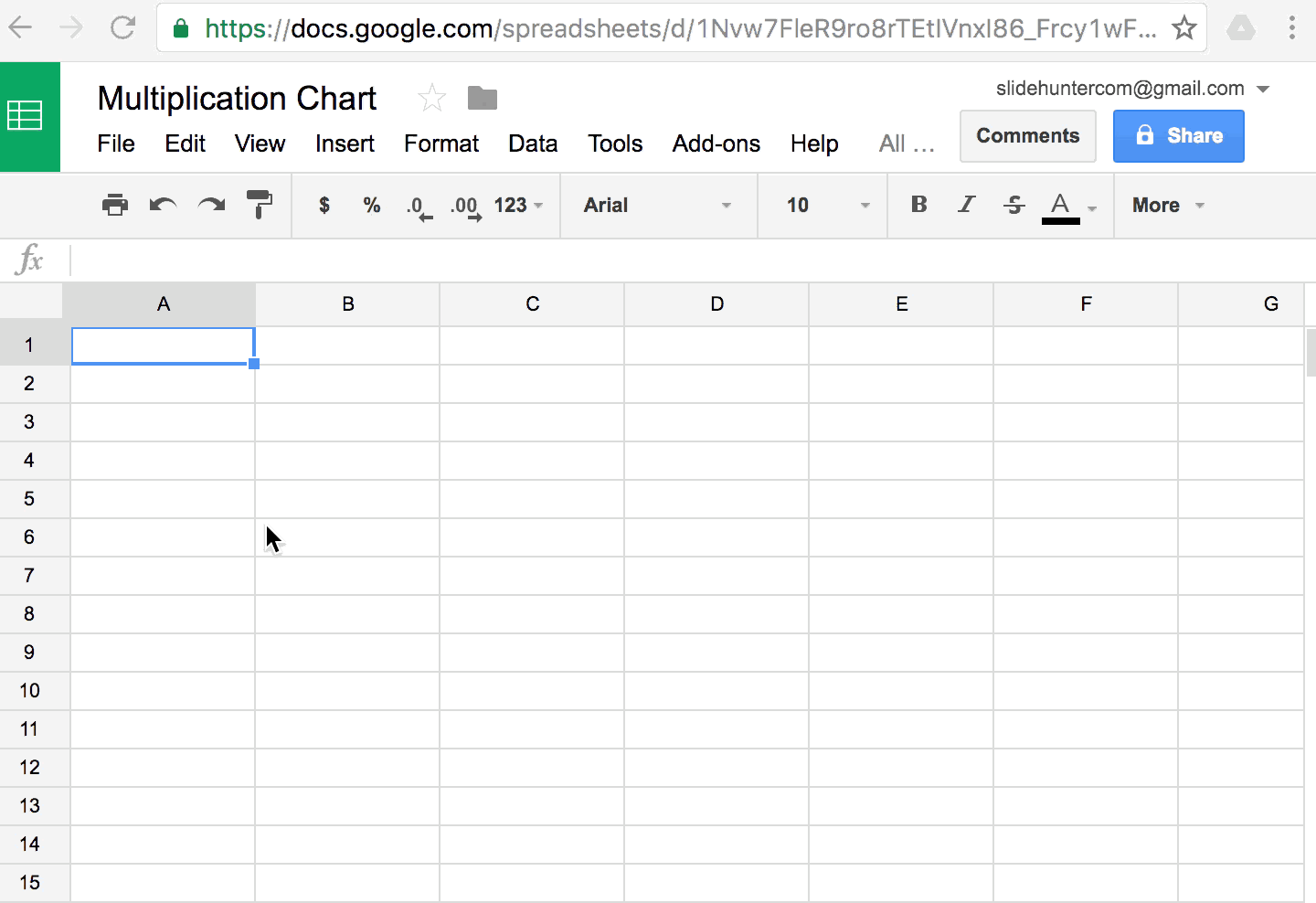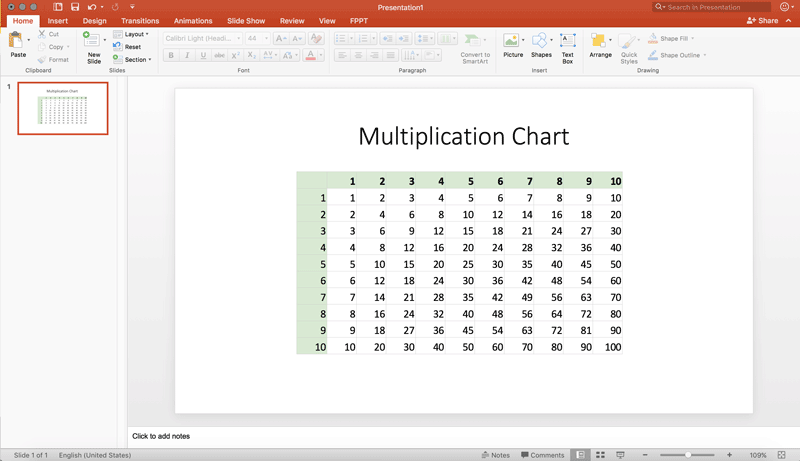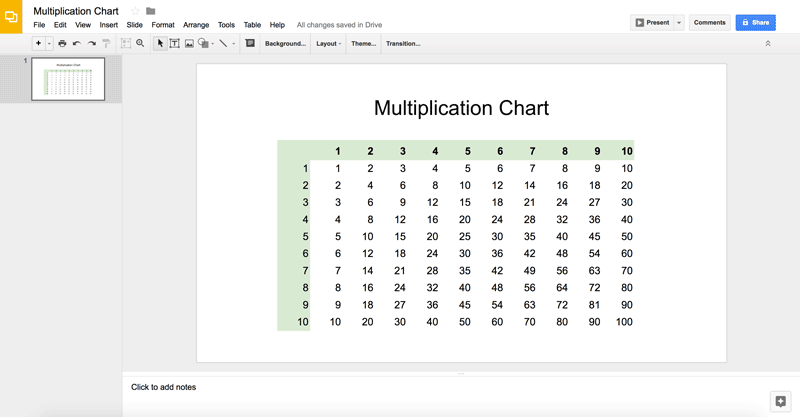Home   Articles   Multiplication Chart for Google Slides & PowerPoint

## Multiplication Chart for Google Slides & PowerPoint

Using Google Sheets we can create a multiplication chart very easy. Then, we can copy the chart to PowerPoint or Google Slides. Here we will show how to make a multiplication chart that can be copied to Microsoft PowerPoint or Google Slides if you want to display a multiplication table chart in your presentations.

A multiplication chart template is very useful if you are making an e-learning presentation or need to teach your students how the multiplication tables work.

### Step 1 – Create a Multiplication Chart Spreadsheet

First step is to create the chart as a spreadsheet, so you can rely on the formulas to build the whole chart very easy. Add 1 to 10 in the columns and then add the 1 to 10 numbers in the rows. For each cell we will add a simple formula that will multiply the numbers in the column by the number in the row.

The formula can be added as follows:As you can see, the first cell will use a formula like:

=\$A2*C\$1

Then, copy the cell horizontally and vertically and finally add some styles to the headers.

Recommended: You can use a free multiplication chart generator to generate a table or multiplication chart easily.

The following screencast will show you how to create this simple multiplication chart using Google Sheets. For this example we are creating a multiplication chart with numbers from 1-100 (also known as a Math multiplication chart 1-100). But using the same approach it could be possible to create a multiplication chart 1-12.### Step 2 – Copy the chart to PowerPoint or Google Slides

Once we have the multiplication chart we can copy it to the slides in PowerPoint and Google Slides.

In PowerPoint, the 10×10 multiplication chart will look as follows:If you prefer to use Google Slides, then the multiplication chart will look as follows: